Question

The hydrolysis of sucrose (C12H22O11) into glucose and fructose in acidic water has a rate constant of 1.8×10−4s−1 at 25 ∘C.Assuming the reaction is first order in sucrose, determine the mass of sucrose that is hydrolyzed when 2.40 L of a 0.140 mol⋅L−1 sucrose solution is allowed to react for 195 minutes.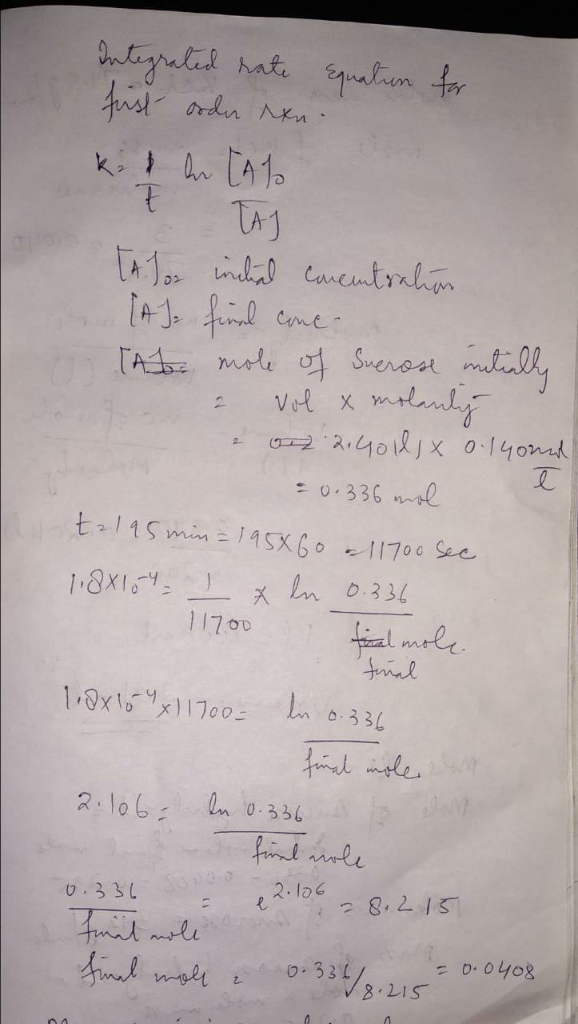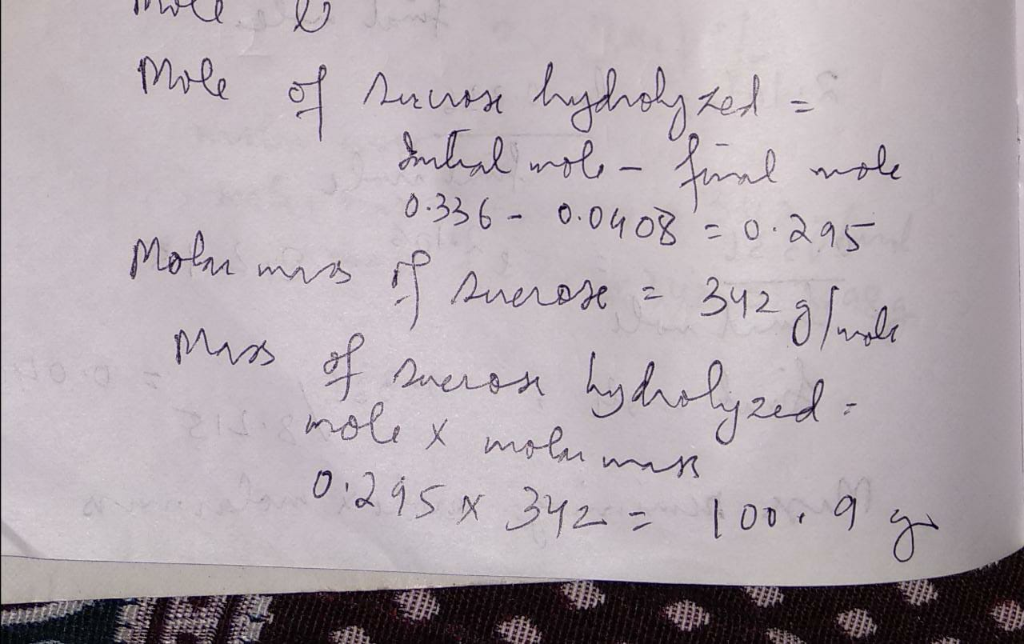#### Earn Coins

Coins can be redeemed for fabulous gifts.

Similar Homework Help Questions
• ### The hydrolysis of sucrose (C12H22O11) into glucose and fructose in acidic water has a rate constant...

The hydrolysis of sucrose (C12H22O11) into glucose and fructose in acidic water has a rate constant of 1.8×10−4s−1 at 25 ∘C. Assuming the reaction is first order in sucrose, determine the mass of sucrose that is hydrolyzed when 2.60 L of a 0.120 M sucrose solution is allowed to react for 195 min.

• ### Assuming the reaction is first order in sucrose, determine the mass of sucrose that is...

The hydrolysis of sucrose into glucose and fructose in acidic water has arate constant of at 25.Assuming the reaction is first order insucrose, determine the mass of sucrose that is hydrolyzed when2.55 of a 0.150 sucrose solution is allowed to react for 200minutes.

• ### Sucrose is hydrolysed in acid medium according to the following reaction: C12H22O11 + H2O -> glucose...

Sucrose is hydrolysed in acid medium according to the following reaction: C12H22O11 + H2O -> glucose + fructose and with a constant of rapidity of 1.8 x 10-4 s-1 at 25oC. What will be the mass of sucrose that is hydrolyzed when 2.55 liters of a solution of initial concentration of 0.150M in sucrose is hydrolysed after 240 minutes? C12H22O11 = 342.34 g / mol

• ### 2) (20 points) Sucrose is readily hydrolyzed to glucose and fructose in acidic solutions. The concentration...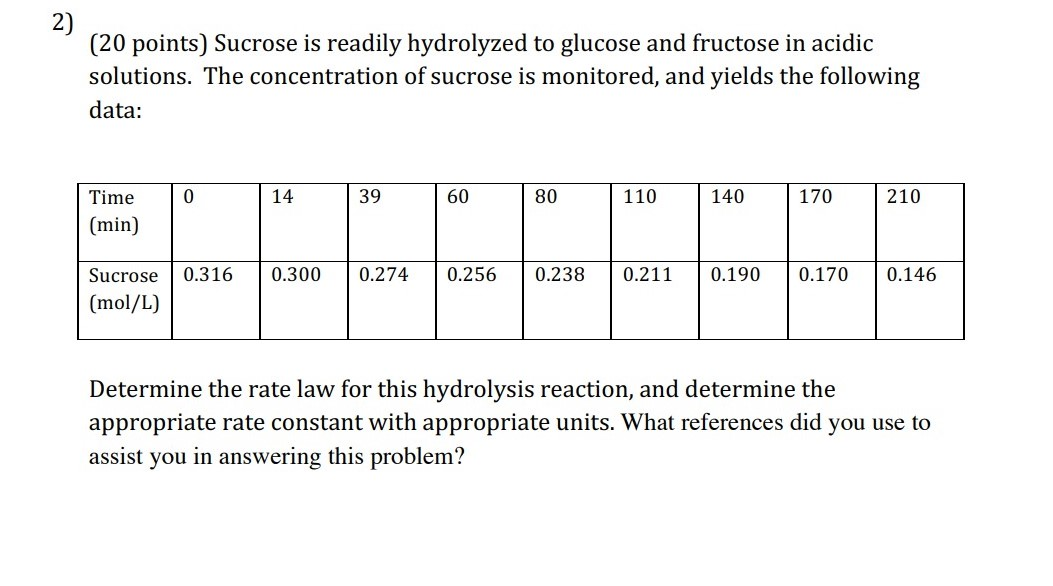2) (20 points) Sucrose is readily hydrolyzed to glucose and fructose in acidic solutions. The concentration of sucrose is monitored, and yields the following data: 0 14 39 60 80 110 140 170 210 Time (min) 0.316 0.300 0.274 0.256 0.238 0.211 0.190 0.170 0.146 Sucrose (mol/L) Determine the rate law for this hydrolysis reaction, and determine the appropriate rate constant with appropriate units. What references did you use to assist you in answering this problem?

• ### The hydrolysis of table sugar (sucrose) occurs by the following overall reaction sucrose + water →...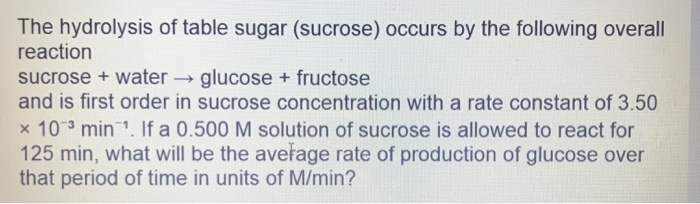The hydrolysis of table sugar (sucrose) occurs by the following overall reaction sucrose + water → glucose + fructose and is first order in sucrose concentration with a rate constant of 3.50 * 10 min?. If a 0.500 M solution of sucrose is allowed to react for 125 min, what will be the average rate of production of glucose over that period of time in units of M/min?

• ### 4. Sucrose or common sugar (C12H22011) is hydrolyzed with water to form fructose (CeH120) and glucose...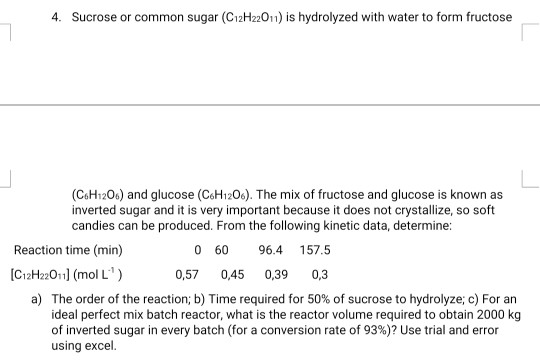4. Sucrose or common sugar (C12H22011) is hydrolyzed with water to form fructose (CeH120) and glucose (CoH1206). The mix of fructose and glucose is known as inverted sugar and it is very important because it does not crystallize, so soft candies can be produced. From the following kinetic data, determine: 0 60 Reaction time (min) 96.4 157.5 [C12H22O11] (mol L.) a) The order of the reaction; b) Time required for 50% of sucrose to hydrolyze; e) For an ideal perfect...

• ### 9- The hydrolysis of Sucrose (Sucrose+ HO change (AG) of- 6.3 Glucose + Fructose) has a...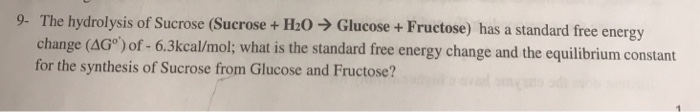9- The hydrolysis of Sucrose (Sucrose+ HO change (AG) of- 6.3 Glucose + Fructose) has a standard free energy kcal/mol; what is the standard free energy change and the equilibrium constant for the synthesis of Sucrose from Glucose and Fructose?

• ### 0 39 1. (13 pts) Sucrose hydrolyses readily to glucose and fructose in acidic solution. An...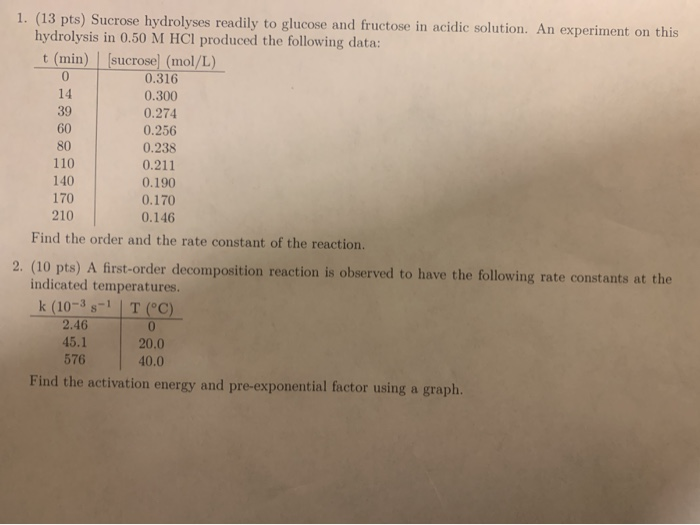0 39 1. (13 pts) Sucrose hydrolyses readily to glucose and fructose in acidic solution. An experiment on this hydrolysis in 0.50 M HCl produced the following data: t (min) (sucrose) (mol/L) 0.316 14 0.300 0.274 0.256 0.238 110 0.211 140 0.190 170 0.170 210 0.146 Find the order and the rate constant of the reaction, 2. (10 pts) A first-order decomposition reaction is observed to have the following rate constants at the indicated temperatures. k (10-3 3-1 | T...

• ### The reaction of sucrose with water in an acidic solution is C12H22O11 + H2O ---> C6H12O6...

The reaction of sucrose with water in an acidic solution is C12H22O11 + H2O ---> C6H12O6 + C6H12O6 The reaction is first order with a rate constant of 6.17 × 10-4 s-1 at 35 °C, when [H+] = 0.10 M. Suppose that in an experiment the initial sucrose concentration was 0.40 M A) What will the concentration be after exactly 4 hours (i.e., 4.00 h)? B) How many minutes will it take for the concentration of sucrose to drop to...

• ### Sucrose, a sugar, decomposes in acidic solution to produce glucose and fructose. The reaction is first...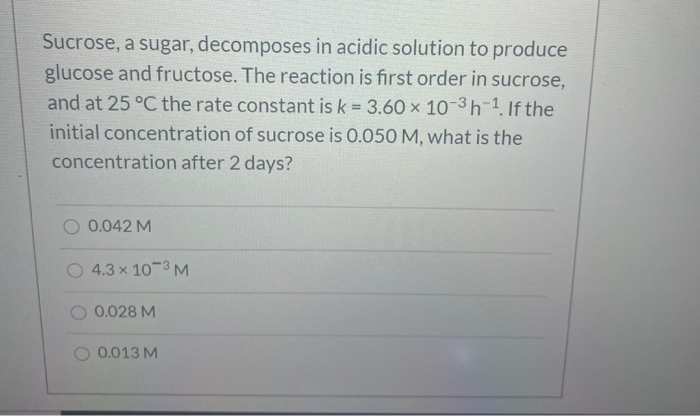Sucrose, a sugar, decomposes in acidic solution to produce glucose and fructose. The reaction is first order in sucrose, and at 25 °C the rate constant is k = 3.60 x 10-3-1. If the initial concentration of sucrose is 0.050 M, what is the concentration after 2 days? O 0.042M 4.3 x 10-3M O 0.028 M 0.013 M